# 9.5. NUMERICAL LINEAR ALGEBRA

This section under major construction.

Java numerics provides a focal point for information on numerical computing in Java.

Linear algebra. Computer science applications: wavelets, transformations in computer graphics, computer vision, Google's PageRank algorithm, linear programming, linear regression, Markov chains. Other applications: linear and nonlinear optimization, control theory, combinatorial optimization, numerical solutions to ODEs, analysis of electrical networks, portfolio optimization, qunatum mechanics. Desire to solve such problems has driven the development of computing technology. BLAS.

Matrix. In numerical linear algebra, a matrix is a rectangular table of real or complex numbers. Given a matrix A, we use the notation Aij to represent the entry in the ith row and the jth column. We can implement a matrix in Java by using a two dimensional array. We access Aij using A[i][j]. We begin indexing at 0 to conform to Java indexing conventions.

Matrix multiplication. The product of two N-by-N matrices A and B is an N-by-N matrix C defined by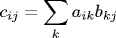The following code fragment computes C = AB.

 for (int i = 0; i < N; i++) for (int j = 0; j < N; j++) for (int k = 0; k < N; k++) C[i][j] += A[i][k] * B[k][j]; 

Row major order vs. column major order. Can have a huge impact on caching and performance. Better to iterate over a row than a column in Java. We can rearrange the matrix multiplication triple loop in any of 3! = 6 ways to achieve the same answer. Each possibility has different memory access patterns may perform very differently (2-3 times) depending on machine architecture since (caching, paging, etc.). Program MatrixMultiplication.java performs matrix multiplication in each of the 6 orderings, and outputs the amount of time it takes. Some architectures have built in gaxpy methods, etc. High performance matrix libraries are very carefully tuned to the machine architecture on which they are to be run to take full advantage of such effects.

Micro-optimizations. Appropriate to consider here since matrix multiplication is the bottleneck computation in many applications. We can explicitly cache certain rows and columns to make the computation faster. We cache row i of A and column j of B. Since Java arrays are row-ordered, we copy the entries in column j of B into a 1D array to facilitate future acceses. Why faster? Better memory access patterns. Avoids bounds checking. Number of assignment statements now proportional to N^2 instead of N^3.

 double[] ai; // row i of A double[] bj = new double[N]; // column j of B for (int j = 0; j < N; j++) { for (int k = 0; k < N; k++) bj[k] = B[k][j]; for (int i = 0; i < N; i++) { ai = A[i]; double s = 0; for (int k = 0; k < N; k++) s += ai[k] * bj[k]; C[i][j] = s; } } 
Could also improve performance (perhaps by up to a factor of 2) by using 1D array of size N2 instead of a Java array of arrays. Here's a good overview of using matrices in Java.

Systems of linear equations. One of the most fundamental and important problems in linear algebra is finding a solution x to the equation Ax = b. Difference equations, interpolation, digital signal processing, least squares, forecasting, Leontief model of economic equilibrium, Hooke's law of elasticity, traffic analysis, temperature equilibrium of heat in a plate, linear and nonlinear optimization.

Kirchoff's voltage law. Loop current analysis of electric circuits.

Row operations. Consider the following system of three linear equations in three unknowns.

  0x0 + 1x1 + 1x2 = 4 2x0 + 4x1 - 2x2 = 2 0x0 + 3x1 + 15x2 = 36 

We can apply a number of identities to transform the system of equations into an equivalent system of equations that is potentially easier to solve. We will use transformation of the following two forms.

• Row interchange. Interchange any two rows. For example, we can interchange the first and second rows above to yield the equivalent system.
  2x0 + 4x1 - 2x2 = 2 0x0 + 1x1 + 1x2 = 4 0x0 + 3x1 + 15x2 = 36 

Swapping rows i and j in a 2D array is an especially efficient operation in Java. We only need to swap the references to the ith and jth rows.

 double[] temp = A[i]; // ith row of A A[i] = A[j]; A[j] = temp; 
• Linear combination. Add or subtract a multiple of one row to another row. For example, we can subtract three times the second equation from the third to obtain yet another equivalent system.
  2x0 + 4x1 - 2x2 = 2 0x0 + 1x1 + 1x2 = 4 0x0 + 0x1 + 12x2 = 24 

This transformation involves multiplication by a real number, so we may end up with real-valued coefficients even if we begin with integer coefficients.

Back-substitution. The last system of equations above is particularly amenable to solution. From the last equation (12 x2 = 24) we can immediately derive x2 = 2. Substituting x2 = 2 into the second equation yields x1 + 2 = 4. Now we can derive x1 = 2. Finally, we can substitute x1 and x2 back into the first equation. This yields 2x0 + 4(2) - 2(2) = 2, which implies x0 = -1. This back-substitution procedure is straightforward to express in Java.

 for (int j = N - 1; j >= 0; j--) { double t = 0.0; for (int k = j + 1; k < N; k++) t += A[j][k] * x[k]; x[j] = (b[j] - t) / A[j][j]; } 

Gaussian elimination. Gaussian elimination is one of the oldest and most widely used algorithms for solving linear systems of equations. The algorithm was explicitly described by Liu Hui in 263 while presenting solutions to the famous Chinese text Jiuzhang suanshu (The Nine Chapters on the Mathematical Art), but was probably discovered much earlier. The name Gaussian elimination arose after Gauss used it to predict the location of celestial objects using his newly discovered method of least squares. Apply row operations to transform original system of equations into an upper triangular system. Then use back-substitution.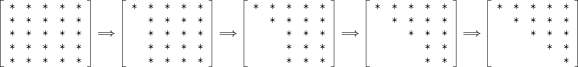The following fantasy code is a straightforward implementation of Gaussian elimination.

 for (int i = 0; i < N; i++) { // pivot within b for (int j = i + 1; j < N; j++) b[j] -= b[i] * A[j][i] / A[i][i]; // pivot within A for (int j = i + 1; j < N; j++) // optimization precompute m = A[j][i] / A[i][i] and loop upwards for (int k = N - 1; k >= i; k--) A[j][k] -= A[i][k] * A[j][i] / A[i][i]; A[j][i] = 0.0; // can stop previous loop at i+1 

Unfortunately, if one of the pivot elements A[i][i] is zero, the code divides by zero and fails spectacularly. There are some important applications where we are guaranteed never to encounter a zero pivot and get stuck (e.g., if matrix is strictly diagonally dominant or symmetric positive definite), but in general, we must ensure that zero pivots never occur by interchanging the row containing the zero pivot with another row beneath it. If no such row exists, then the system either has no solution or it has infinitely many. (See Exercises XYZ and XYZ.)

Partial pivoting. One common pivot strategy is to select the row that has the largest (in absolute value) pivot element, and do this interchange before each pivot, regardless of whether we encounter a potential zero pivot. Program GaussianElimination.java implements Gaussian elimination with partial pivoting. This selection rule is known as partial pivoting. It is widely used because, in addition to fixing the zero pivot problem, it dramatiaclly improves the numerical stability of the algorithm. To see its effects, consider the following system of equations, where a = 10-17.

 ax0 + x1 = 1 x0 + 2x1 = 3 

If we don't pivot on the largest coefficient, then Gaussian elimination produces the solution (x0, x1 = (0.0, 1.0), whereas Gaussian elimination with partial pivoting yields (1.0, 1.0). The exact answer is (99999999999999997/99999999999999998, 50000000000000000/49999999999999999). The solution with partial pivoting yields 16 decimal digits of accuracy while the one without partial pivoting has 0 digits of accuracy for x0. Although this example was contrived to demonstrate and magnify the effect, such situation do arise in practice. This example is a situation where the problem instance is well-conditioned, but the algorithm (without partial pivoting) is unstable. In this example, the potential problem is resolved by using partial pivoting. (See Exercise XYZ for an example where partial pivoting fails even though the intance is not ill-conditioned.) Numerical analysts use Gaussian elimination with partial pivoting with high confidence even though it is not provably stable. When the problem instance itself is ill-conditioned, no floating point algorithm will be able to rescue it. To detect such cases, we compute the condition number, which measures how ill-conditioned a matrix is. # bits in solution ~ # bits in data - lg kappa(A)

Complete pivoting. Choose pivot element to be the one with largest absolute value among entries that still need to be row-reduced. Swap both rows and columns (instead of just rows). More bookkeeping and time searching for the pivot, but better stability. However, scientists rarely use complete pivoting in practice because partial pivoting almost always succeeds.

Gaussian elimination can also be used to compute the rank since row operations do not change the rank. Rank of an m-by-n matrix: if you get stuck when pivoting in column j, continue to column j+1. Rank = # nonzero rows upon termination.

interesting matrices for testing.

Iterative methods. Roundoff error can accumulate in Gaussian elimination. Iterative methods (Gauss-Seidel, Jacobi iteration, successive over relaxation) can also be used to refine solutions to linear systems of equations obtained via Gaussian elimination. Also can solve from scratch - a big advantage if A is sparse. Gauss Seidel: x0 = b, xk+1 = (I - A)xk + b. If all diagonal of entries of A are 1 (can assume by rescaling) and all eigenvalues of (I - A) are less than 1 in magnitude, then Gauss-Seidel iterates converge to true solution.

 public class Matrix { private double[][] data; private int M; private int N; } 

The program Matrix.java implements the following operations: add, multiply, transpose, identity matrix, trace, and random matrix. More ops: inverse, rank, determinant, eigenvalues, eigenvectors, norm, solve, condition number, singular values.

Java libraries for numerical linear algebra. Building efficient and robust algorithm for linear algebraic problems is a challenging task. Fortunately, such algorithms have been refined over the past few decades, and mature libraries are available and easy to access. JAMA: A Java Matrix Package is such a library for matrix operations including solving Ax = b, computing eigenvalues, computing the singular value decomposition, etc. The algorithms are the same as the ones in EISPACK, LINPACK, and MATLAB. This software has been released into the public domain by The MathWorks and the National Institute of Standards and Technology (NIST). Here is the Javadoc documentation. Program JamaTest.java illustrates how to interface with this library. It solves a system of 3 linear equations in 3 unknowns using the JAMA package.

Eigenvalues and eigenvectors. Given a square matrix A, the eigenvalue problem is to find solutions to Ax = λx. A scalar λ that satisfies this equation is called an eigenvalue and the corresponding vector x is called an eigenvector. Solutions to the eigenvalue problem play an important role of the computational infrastructure in many scientific and engineering disciplines. In 1940, Tacoma Narrows Bridge collapsed four months after it was built because frequency of wind was too close to natural frequency of bridge, and this caused overwhelming oscillations. Natural frequency of the bridge is the smallest eigenvalue in linear system that models the bridge. Eigenvalues also used to analyze modes of vibrations of a string, solutions to differential equations, Leslie matrix model of population dynamics, test for cracks or deformities in a solid, probe land for oil, damp noise in car passenger compartment, design concert halls for optimum sound quality, compute axes of inertia of a rigid body.

Spectral decomposition. If A is symmetric, then the eigenvalue decomposition is A = VΛVT where Λ is the diagonal matrix of eigenvalues and V is the orthogonal matrix of eigenvectors. Program Eigenvalues.java generates a random symmetric positive definite matrix and computes its spectral decomposition using the Jama library EigenvalueDecomposition.

Power method. In many science and engineering applications, the principal eigenvalue (largest in absolute value) and associated eigenvector reveal the dominant mode of behavior. For example, Google uses it to rank the most important web pages, a structural engineer uses it to measure the maximum load of a bridge, a sound engineer uses it to measure the lowest resonating frequency in a concert hall. The power method is a simple scheme to isolate the largest eigenvalue and the associated eigenvector.

• x = Ax
• x = x / |x|
• λ = xTAx / xTx

Here |x| means the L1 norm (for probability distributions) or L2 norm. Under general technical conditions, λ converges to the principal eigenvalue and x converges to the principal eigenvector.

Markov chain stationary distribution. A Markov chain is.... Like a randomized NFA. Compute the fraction of time that the Markov chain spends in each state. Stationary distribution satisfies π A = π. The vector π is the (normalized) eigenvector corresponding to the eigenvalue 1. Under certain technical conditions (ergodic), the stationary distribution is unique and it is the principle eigenvector of AT. All components of this eigenvector are guaranteed to be nonnegative.

Google's PageRank algorithm. Use eigenvalues to rank importance of web pages or rank football teams according to strength of schedule. good discussion.

Jack Dongarra has an online guide Templates for the Solution of Algebraic Eigenvalue Problems.

Singular values. The singular value decomposition is a fundamental concept in science and engineering, and one of the most central problems in numerical linear algebra. Given an M-by-N matrix A, the singular value decomposition is A = UΣVT, where U is an M-by-N matrix with orthogonal columns, Σ is an N-by-N diagonal matrix, and V is an N-by-N orthogonal matrix. It is also known as principal component analysis (PCA) in statistics and the Karhunen-Loeve or Hotelling expansion in pattern recognition. The SVD is well-defined for any M-by-N matrix (even if the matrix does not have full row or column rank) and is essentially unique (assuming the singular values are in descending order). It is efficiently computable in time O( min { MN^2, M^2N } ). It has many astonishing and beautiful properties, which we will only begin to explore. The SVD has many applications: multiple linear regression, factor analysis, computer graphics, face recognition, noise reduction, information retrieval, robotics, gene expression analysis, computational tomography, geophysical inversion (seismology), image compression, image deblurring, face recognition, using optics linear sensitivity matrices to analyze spacecraft dynamics, visualization of chemical databases, and latent semantic indexing (LSI). Also widely used in biology to deconvolute a titration involving a mixture of three pH indicators, in protein dynamics to analyze the movement of myoglobin, analysis of microarray data, reverse engineering gene networks.

Program SVD.java computes the singular values of a random 8-by-5 matrix. It also prints out the condition number, numerical rank, and 2-norm.

Image processing. Lossy compressions. A popular technique for compressing images is other data is via the SVD or Karhunen-Loeve decomposition. We can treat an M-by-N image of pixels, as three M-by-N arrays red, green, and blue intensities, each intensity is between 0 and 255. Using the SVD, we can compute the "best" rank r approximation to each of the three matrices. This can be stored using only r(M + N + 1) values instead of MN. As r gets larger, the quality of the image improves, but at the expense of more storage.

One of the SVDs most important properties is that the truncated SVD Ar = UrSrVr is the best rank r approximation to A, where Ur denotes the first r columns of U, Vr denotes the first r columns of V, and Sr denotes the first r rows and columns of S. Here "best" means L_2 norm - Ar minimizes sum of the squares of the differences between A and Ar.

Program KarhunenLoeve.java reads in a picture and an integer r, compute the best rank r approximation of each of its red, green, and blue matrices, and display the resulting compressed picture. The key subroutine computes the best rank r approximation to matrix A. The method getMatrix(i1, i2, j1, j2) returns the submatrix of A bounded by the specified row and column indices.

 public static Matrix KL(Matrix A, int r) { int M = A.getRowDimension(); int N = A.getColumnDimension(); SingularValueDecomposition svd = A.svd(); Matrix Ur = svd.getU().getMatrix(0, M-1, 0, r-1); Matrix Vr = svd.getV().getMatrix(0, N-1, 0, r-1); Matrix Sr = svd.getS().getMatrix(0, r-1, 0, r-1); return Ur.times(Sr).times(Vr.transpose()); } 

The images below give the results of the KL-transform on the famous Mandrill test image for ranks 2, 5, 10, 25, 50, and 298. The last one is the original image.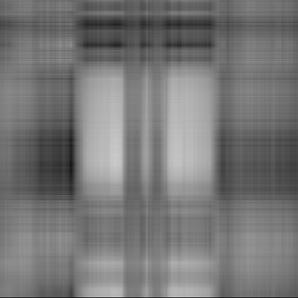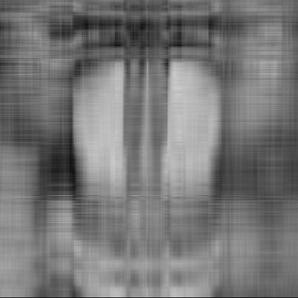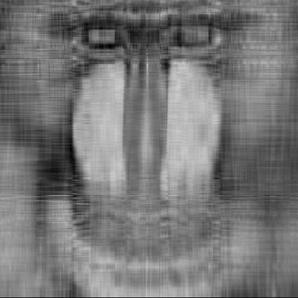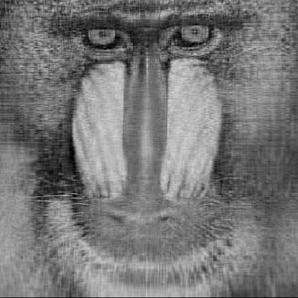Latent semantic indexing. LSI used by Google to classify web pages, linguists to classify documents, etc. Create matrix where rows index terms in a document and column index documents. The matrix entry (i, j) is some function of how many times the term i appears in document j. The matrix AA^T measure the document similarities. Eigenvectors correspond to linguistic concepts, e.g., sports might subsume the terms football, hockey, and baseball. LSI techniques can identify hidden correlations between documents, even if the documents don't share any terms in common. For example, the terms car and automobile get pulled together, since both occur frequently with the terms tire, radiator and cylinder.

Hubs and authorities. Kleinberg's method for finding relevant web pages. Aij = 1 if there is a link from i to j, and 0 otherwise. The matrix ATA counts how many links i and j have in common; the matrix AAT counts how many common pages link to i and j. A hub is a page that points to multiple authoritative pages; an authority is a page that is pointed to by multiple hubs. The principal component of ATA (or equivalently the first column of U in the SVD A = USVT) gives the "principle hubs"; the principle component of AAT (or equivalently the first column of V) gives the "principle authorities."

Gene expression data analysis. Subject genes to a battery of experiments and try to cluster genes with similar responses together. Group genes by transcription response, grouping assays by expression profile.

Sparse matrices. An N-by-N matrix is sparse if the number of nonzeros is proportional to N. Sparse matrices of dimension 100,000 arise in optimization and solutions to partial differential equations. The search engine Google computes with a monstrous sparse matrix of size N = 4 billion. The 2D array representation is rendered useless in these contexts. For example, to compute a matrix-vector product would require quadratic space and time. The power method for computing the principal eigenvector requires a fast matrix-vector multiply. We will describe how to do the same computation in linear time. The main idea is to explicitly store only the s nonzeros of the matrix A, while retaining enough auxiliary information to compute with A. We will describe one popular sparse matrix storage scheme known as compressed row storage. We store all s nonzero entries consecutively in a one dimensional array val[] so that val[j] stores the jth nonzero (in the order from left to right, and top to bottom). We also maintain two extra auxiliary arrays to provide access to the individual matrix entries. Specifically we maintain one integer array col[] of size s such that col[j] is the column in which the jth nonzero appears. Finally, maintain an integer array row of size N+1 such that row[i] is the index of the first nonzero from row i in the array val. By convention row[N] = s.

 0.1 0.0 0.0 0.2 val[] = 0.1 0.2 0.3 0.4 0.5 0.3 0.0 0.0 0.0 col[] = 0 3 0 1 2 0.0 0.0 0.0 0.0 0.0 0.4 0.5 0.0 row[] = 0 2 3 3 5 

Since each double consumes 8 bytes and each int consumes 4 bytes, the overall storage for CRS is roughly 12s + 4N. This compares favorably with the 8N2 bytes required with the 2D array representation. Now, if A is represented using CRS, then the matrix-vector product y = Ax is efficiently computed using the following compact code fragment. The number of floating point operations is now proportional to (s + N) instead of N2.

 double[] y = new double[N]; for (int i = 0; i < N; i++) for (j = row[i]; j < row[i+1]; j++) y[i] += val[j] * x[col[j]]; 

Computing y = ATx is a little trickier since the naive approach involves traversing the columns of A, which is not convenient using CRS format. Switching the order of summation yields:

 double[] y = new double[N]; for (int j = 0; j < N; j++) for (int i = row[j]; j < row[j+1]; i++) y[col[i]] += val[i] * x[j]; 

Conjugate gradient method. A Krylov-subspace method when A is symmetric positive definite. [Probably omit or leave as an exercise.]

#### Q & A

Q. Is there ever a reason to explicitly compute the inverse of a matrix?

A. Yes, if it's asked for on an exam. In practice, it's almost never necessary. To solve Ax = b, you should use Gaussian elimination instead of forming A-1b. If you need to solve Ax = b for many different values of b, then use something called the LU decomposition. It's twice as fast and has better numerical accuracy and stability properties.

#### Exercises

1. Add a method frobenius() to Matrix that returns the Frobenius norm of the matrix. The Frobenius norm is the square root of sum of the squares of all the entries.
2. Add a method normInfinity that returns the infinity norm of the matrix. The infinity norm (a.k.a., row sum norm) is the maximum sum obtained by adding the absolute values of the elements in each row.
3. Add a method trace that returns the trace of the matrix. The trace is the sum of the diagonal entries.
4. Add a method isSymmetric that returns true if the matrix is symmetric and false otherwise. The matrix A is symmetric if it is square and Aij = Aji for all i and j.
5. Add a method isTridiagonal that returns true if the matrix is tridiagonal, and false otherwise.
6. Given an N-by-N array a[][], write a code fragment to transpose a in-place. That is, use at most a few extra variables of storage.
7. Add a method plusEquals that takes a Matrix B as input and over-writes the invoking matrix with the sum of itself and B.
8. Suppose you run Gaussian elimination without exchanging rows. Show that it will fail on
 0x0 + x1 = 7 x0 + 0x1 = 5 

9. Alternative pivot strategy: choose the row in column j such that |A_ij| / max_k | A_ik| is as large as possible.
10. Solve the following system Ax = b by hand using partial pivoting. Creates numbers as big as 2^5. Generalizes to 2^N for N-by-N system.
  1 0 0 0 1 1 1/2 -1 1 0 0 1 0 0 A = -1 -1 1 0 1 b = 0 x = 0 -1 -1 -1 1 1 0 0 -1 -1 -1 -1 1 0 1/2 
Intermediate expression swell.
11. Consider an N-by-N matrix A of the following form for N = 100,
  1.1000 0 0 0 0 1.0000 -0.9000 1.1000 0 0 0 1.0000 -0.9000 -0.9000 1.1000 0 0 1.0000 -0.9000 -0.9000 -0.9000 1.1000 0 1.0000 -0.9000 -0.9000 -0.9000 -0.9000 1.1000 1.0000 -0.9000 -0.9000 -0.9000 -0.9000 -0.9000 1.0000 
and a vector b = [1, 0, 0, ..., 0, 0]. The matrix A is nonsingular and Ax = b has the unique solution x = [1/2, 0, 0, ..., 0, 9/20]. Solve Ax = b using Gaussian elimination with partial pivoting (either our code or the Jama library). Examine the residual error and observe that the solution vector has no significant digits of accuracy in many coordinates. The matrix A is well-conditioned, so it is not a result of the problem being ill-conditioned as in XYZ. Instead, it is because partial pivoting is unstable, and this input highlights this defect. Name your program PartialPivotStability.java.
12. Solve the following upper triangular linear system of equations by hand using back-substitution.
  2x1 + 4x2 - 2x3 = 2 0x1 + 1x2 + 1x3 = 4 0x1 + 0x2 + 4x3 = 8 

13. Solve the following linear system of equations by hand using Gaussian elimination and back-substitution.
  2x1 + 4x2 - 2x3 = 2 4x1 + 9x2 - 3x3 = 8 - 2x1 - 3x2 + 7x3 = 10 

Answer: -1 2 2. You should obtain the upper triangular system in the previous exercise along the way.

14. Solve the following linear system of equations.
 -9x1 - x2 + x3 + x4 + x5 + 3x6 = 2 2x1 - 7x2 - x3 + x4 + x5 + x6 = -12 x1 + 2x2 - 9x3 - x4 + x5 + 3x6 = -33 x1 + x2 + 2x3 - 7x4 - x5 + x6 = -29 x1 + x2 + x3 + 2x4 - 9x5 - 3x6 = 21 x1 + x2 + x3 + x4 + 2x5 - 7x6 = -13 

Answer: 2 3 5 7 -1 4.

Area of a triangle and ccw. Formulas for the area of a triangle have been known for 2000 years. Grade school formula (1/2 base * height) and Heron's formula require analyzing trigonometric functions or taking square roots. In the 17th century Descartes and Fermat used linear algebra to gain new insight into geometric problems. For example, the following determinant gives twice the signed area of the triangle with vertices a, b, and c.

 | ax ay 1 | | bx by 1 | | cx cy 1 | 
The sign of the determinant specifies whether or not c is to the left or, to the right of, or on the line directed from a to b. This ccw test is a useful primitive for convex hull and other computational geometry algorithms. Generalizes naturally to higher dimensions for tetrahedron and other simplices.

In-circle test. Determine if the point d lies inside or outside the circle defined by the three points a, b, and c in the plane. Application: primitive operation in Delaunay triangulation algorithms. Assuming that a, b, c are labeled in counterclockwise order around the circle, the following determinant is positive if d is inside the circle, negative if d is outside the circle, and zero if all four points are cocircular. This generalizes naturally to higher dimensions, e.g., point inside a sphere defined by 4 points.

 | ax ay ax^2 + ay^2 1 | | bx by bx^2 + by^2 1 | | cx cy cx^2 + cy^2 1 | | dx dy dx^2 + dy^2 1 | 
15. Given the equation of a plane ax + by + cz = 1 that contains the three points (10, 5, 2), (3, 8, 9), and (3, 6, -1).
16. Find the eigenvalues and eigenvectors of the following matrix.
  3 -1 -1 3 

Answer: λ1 = 2, λ2 = 4.

17. Suppose you are presented with the problem of computing cTA-1d. Explain how to do it without explicitly computing A-1. Solution. Solve Ax = d for x using Gaussian elimination. Now the desired answer is cTx. Whenever you see an inverse in a formula, always think of it as solving an equation rather than computing an inverse.

#### Creative Exercises

1. Complex matrices. Create an abstract data type to represent complex matrices.
2. Lights out. Implement a solver for the games Lights Out. Solve a linear system of equations (over Z_2) to determine which lights to turn on (if such a solution exists).
3. Feasibility detection. Can't find a non zero pivot and the current right hand side is nonzero.
4. Certificate of infeasibility. If there is no solution to Ax = b, then Gaussian elimination will fail. If so, then there exists a vector c such that cTA = 0 and cTb ≠ 0. Modify Gaussian elimination so that it produces such a vector when Ax = b has no solutions.
5. Tridiagonal matrices. Implement a data type TridiagonalMatrix that implements a tridiagonal matrix using three 1-D arrays. Design an algorithm that solves Ax = b when A is a square tridiagonal matrix. Your algorithm should run in linear time.
6. Strassen's algorithm. N2.81 divide-and-conquer algorithm for matrix multiplication. Compare vs. Gaussian elimination.
7. Special matrices. Create classes DiagonalMatrix, TridiagonalMatrix using inheritance and override the methods for solving a linear system of equations and matrix multiplication....
8. Markov chains. Markov chains are a simple mathematical tool for modeling behavioral patterns. Widely used in many scientific areas including queuing theory, statistics, modeling population processes, and gene prediction. Glass and Hall (1949) distinguished 7 states in their social mobility study:
2. Managerial
3. Inspectional, supervisory, non-manual high grade
5. Skilled manual
6. Semi-skilled manual
7. Unskilled manual

The table below presents the data from their study. Entry (i, j) is the probability of transitioning from state i to j.

  { 0.386, 0.147, 0.202, 0.062, 0.140, 0.047, 0.016 } { 0.107, 0.267, 0.227, 0.120, 0.207, 0.052, 0.020 } { 0.035, 0.101, 0.188, 0.191, 0.357, 0.067, 0.061 } { 0.021, 0.039, 0.112, 0.212, 0.431, 0.124, 0.061 } { 0.009, 0.024, 0.075, 0.123, 0.473, 0.171, 0.125 } { 0.000, 0.103, 0.041, 0.088, 0.391, 0.312, 0.155 } { 0.000, 0.008, 0.036, 0.083, 0.364, 0.235, 0.274 } 

Write a program MarkovChain.java to compute some interesting quantity.

9. Hilbert matrix. Write a program Hilbert.java that reads in a command line parameter N, creates an N-by-N Hilbert matrix H, numerically computes its inverse H-1. The i-j entry of a Hilbert matrix is 1/(i+j-1). All Hilbert matrices are invertible. Below is the 4-by-4 Hilbert matrix and its inverse
  1 1/2 1/3 1/4 16 -120 240 -140 H = 1/2 1/3 1/4 1/5 H^-1 = -120 1200 -2700 1680 1/3 1/4 1/5 1/6 240 -2700 6480 -4200 1/4 1/5 1/6 1/7 -140 1680 -4200 2800 

What happens when you try to invert a 100-by-100 Hilbert matrix?

Answer: Jama matrix inverter reports that the matrix is not invertible. The Hilbert matrix is ill-conditioned, and most linear algebra packages have difficulty inverting this matrix for sufficiently large N. Note that although it inverts smaller matrices without reporting an error, there is substantial error in the results.

10. Markov chain stationary distribution. If Markov chain M is irreducible (possible to get from any state to any other state after finitely many transitions) and aperiodic, then it it called ergodic. The stationary distribution is the (unique) eigenvector of M corresponding to eigenvalue = 1.
11. Detailed balance. A special case of an ergodic Markov chain is one that satisfies detailed balance: there exists πi such that πi pij = πj pji for all i and j != i. Show that if detailed balance is satisfied, then π P = π. Hint: sum over j.
12. Random walk on a ring. Suppose you have a circle of N nodes and you start at node 1. At each step, you flip a fair coin and go clockwise or counterclockwise accordingly. Calculate the probability that each vertex (other than 1) is the last one visited. Solution: all have equal likelihood of being last!
13. Leontief input-output model. Branch of economics that uses linear algebra to model interdependencies of industries. (Wassily Leontief won 1973 Nobel Prize in Economics) description. Leontief divided American economy into 81 sectors ( petroleum, textiles, transportation, chemicals, steel, agriculture, etc.) example Technology matrix represents amount of each resource required to produce one unit of another resource. For example, producing 1 unit of petroleum requires 0.2 units of transportation, 0.4 units of chemicals, and 0.1 unit of itself. Units measured in millions of dollars.
 Petroleum 0.10 0.40 0.60 0.20 Textiles 0.00 0.10 0.00 0.10 Transportation 0.20 0.15 0.10 0.30 Chemicals 0.40 0.30 0.25 0.20 
If economy produces net of 900 milion dollars of petroleum, 300, 850, and 800 of textiles, transportation, and chemicals, what is amount of each consumed internally by economy. Multiply Ax to get b [880, 110, 550, 822.50].
14. Leontief closed model. Balanced ecomomy: Ax = x. Total production of each sector equals total consumption.
15. Leontief open model. External demand vector d: Ax + d = x Matrix is productive if solution exists which has nonnegative components. \$1 coal requires 0.3 electricity, 0.1 auto, and 0.1 of itself.
 Coal 0.10 0.25 0.20 Electricity 0.30 0.40 0.50 Auto manufacturing 0.10 0.15 0.10 
Demand d = [50 75 125]. (I - A)x = d. x = [229.9, 437.8, 237.4].
16. Leslie matrix model. In population ecology, the Leslie matrix models the age distribution of a population this is naturally segmented into age classes. Let Fi be the fecundity rate of females in class i, and let Si be the survival rate from class i to i+1. The Leslie matrix L is constructed by: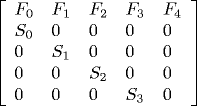The follow table lists the birth and survival probabilities for female New Zealand sheep. The original source is: [from G. Caughley, "Parameters for Seasonally Breeding Populations," Ecology 48(1967)834-839].

Age (years) Birth rate Survival rate
0-1 0.000 0.845
1-2 0.045 0.975
2-3 0.391 0.965
3-4 0.472 0.950
4-5 0.484 0.926
5-6 0.546 0.895
6-7 0.543 0.850
7-8 0.502 0.786
8-9 0.468 0.691
9-10 0.459 0.561
10-11 0.433 0.370
11-12 0.421 0.000
Leslie matrix property: unique positive eigenvalue and corresponding eigenvector has all entries real and of the same sign. In this example 1.175. Use eigenvalues to analyze.
17. Leslie matrix model. Chinook salmon in Columbia River Basin (Kareiva et al, 2002)
 eggs per yearling 4 eggs per 2 year old 20 eggs per 3 year old 60 survival rate of eggs = 0.005 yearling survival 0.3 2 year old survival 0.6 0 4 20 60 0.05 0 0 0 0 0.3 0 0 0 0 0.6 0 
Principal eigenvalue = rate of population growth = 0.93 (decrease 7% per year). Stationary distribution.
18. Maximum cardinality matching. Given a bipartite G graph with N vertices on each side, find a matching of maximum cardinality. Form N-by-N adjacency matrix. If there is an edge between i and j, set entry i-j to a random number between 0 and 2N. If determinant is nonzero, then G has a perfect matching. If determinant is zero, then with probability at least 1/2, G does not have a a perfect matching. Repeat to get better error tolerance. Same idea to get max cardinality, but use rank(G). Note: there are faster algorithms for finding a perfect matching using graphs, but this one is efficiently parallelizable.
19. Maximum cardinality matching. Redo previous exercise but to avoid overflow to all computations mod p, where p is a prime between N and 2N.
20. Maximum cardinality matching. Devise an algorithm to find the perfect matching if it exists.
21. Number of paths from s to t. Given an undirected graph G, count the number of paths from s to t. Look at the kth power of the adjacency matrix G. Note that paths need not be simple.
22. Finding all simplicial vertices of a graph. Given an undirected graph G, a simplicial vertex is a vertex v such that if you take any two of its neighbors x and y, then there is an edge between x and y. Find all simplicial vertices in a graph with N vertices in time less than N3. Hint: compute the N-by-N vertex adjacency matrix A, where Avw = 1 if there is an edge between v and w or if v = w, and 0 otherwise. Fact: a vertex is simplicial if and only if (A2)vv = (A2)vw for all vertices w adjacent to v. Use fast matrix multiplication to compute A2.
23. Counting the number of triangles in a directed graph. Given a directed graph G, a triangle is three vertices x, y, and z such that there is a directed cycle x->y->z->x or x->z->y->x. Given a graph with N vertices, write a program to print out all triangles in time less than N3. Hint: compute the N-by-N vertex adjacency matrix A, where Avw = 1 if there is an edge from v to w, and 0 otherwise (including if v = w). Then (A2)vw is the number of paths going from v to w through exactly one other intermediate vertex. There is a triangle x->y->z if an only if (A2)xz > 0 and Azx = 1.
24. Numerical rank. The numerical rank is the number of nonzero singular values. Use SVD to compute.
25. Condition number. The condition number κ is the ratio of the largest singular value to the smallest one. First proposed by Alan Turing! For a square matrix, it measures how close the matrix is to being singular. For a rectangular matrix, it measures how close the matrix is to being rank deficient. It is also useful to bound how much the solution to Ax = b can change if we make a small perturbation b. If the condition number is very large then we don't expect very accurate results when we solve Ax = b. Roughly speaking the number of bits of accuracy in the solution is equal to the number of bits of accuracy in the input minus log2κ.

Here's a particularly pathological 4-by-4 matrix whose condition number is around 10^65. This means that will less than 65 decimal digits of precision, we cannot expect any significant digits of accuracy in our answer. [Reference: S.M. Rump. A Class of Arbitrarily Ill-conditioned Floating-Point Matrices. SIAM Journal on Matrix Analysis and Applications (SIMAX), 12(4):645-653, 1991.]

  -5046135670319638 -3871391041510136 -5206336348183639 -6745986988231149 -640032173419322 8694411469684959 -564323984386760 -2807912511823001 -16935782447203334 -18752427538303772 -8188807358110413 -14820968618548534 -1069537498856711 -14079150289610606 7074216604373039 7257960283978710 
26. Symmetric positive definite. An N-by-N matrix A is symmetric positive definite if it is symmetric and xTAx > 0 for all x ≠ 0 (equivalently, all eigenvalues are positive). Symmetric positive definite matrices arise as covariance matrices in statistics, stiffness matrices in finite element methods, normal equations in linear regression. Write a program to test whether a matrix is symmetric positive definite. Hint: all pivots are positive in Gaussian elimination (and no row interchanges).
27. Cholesky decomposition. Write a program Cholesky.java to compute the Cholesky decomposition of a symmetric positive definite matrices: A = LLT. Use the Cholesky-Banachiewicz algorithm.
 for (int i = 0; i < N; i++) { for (int j = 0; j <= i; j++) { double sum = 0.0; for (int k = 0; k < j; k++) { sum += L[i][k] * L[j][k]; } if (i == j) L[i][i] = Math.sqrt(A[i][i] - sum); else L[i][j] = 1.0 / L[j][j] * (A[i][j] - sum) } } 
28. Multidimensional scaling. Reconstruct relative point positions given only inter-point distances. Solution to fundamental problem is technique used to draw map of US in 3d over surface of globe, etc. Given N points in the two dimensional plane, define an N-by-N matrix A such that Aij is the Euclidean distance between point i and point j. Find an isometric embedding, i.e., a set of points that satisfy these distances. Hint: let I be the N-by-N identity matrix, let B be the N-by-N matrix of squared pairwise distances, and let u be an N-by-1 vector of all 1's, B be the matrix of squared distances among the n points, and let C = (-1/2)(I - uuT/N) * B * (I - uuT/N). If the points lie in an m-dimensional space, then C is positive semidefinite and has rank m. Let C = LLT be the Cholesky decomposition of C. Then L contains the coordinates of the points.
29. Compressed column storage. Compressed column storage (a.k.a. Harwell-Boeing storage) is completely analogous to compressed row storage except that the columns are stored sequentially. Implement matrix-vector multiply when using CCS. Hint: storing A using CCS is the same as storing AT using CRS.
30. Sparse band matrices. Use compressed diagonal storage to represent a banded matrix. Implement matrix-vector multiplication efficiently.
31. Roots of a polynomial. Given a polynomial anxn + ... + a1x + a0, we can compute its roots by finding the eigenvalues of the companion matrix.Not numerically stable, so it's not guaranteed to work reliably.
32. Real roots of a polynomial. Write a program RouthHurwitz.java that takes as input the positive, real, coefficients of a polynomial a0 + a1x1 + ... + anxN and determines whether all zeros of the polynomial have negative real part. This is a classic problem in control theory and can be used to determine whether a linear system is stable (if the real parts of every root is negative then it is stable; otherwise unstable). From the celebrated Routh-Hurwitz stability criterion this is true if and only if all principal subdeterminants of the following N-by-N matrix are strictly positive.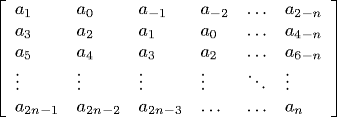By convention, am = 0 if m < 0 or m > n.

Solution: to compute all subdeterminants, run GE without pivoting - if you encoutner any zero or negative pivots, answer no. We could compute eigenvalues as in the previous exercise, but it can be done without explicitly computing the roots.

33. Graph connectivity. Given adjacency matrix of undirected graph A(G), the multiplicity of the largest eigenvalue (lambda = 1), equals the number of connected components in G. G is bipartite iff -1 is an eigenvalue of A(G).